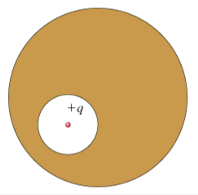# Problem: A spherical cavity is hollowed out of the interior of a neutral conducting sphere. At the center of the cavity is a point charge, of positive charge q.(a) What is the total surface charge qint on the interior surface of the conductor (i.e., on the wall of the cavity)? (b) What is the total surface charge qext on the exterior surface of the conductor?(c) What is the magnitude Eint of the electric field inside the cavity as a function of the distance r from the point charge?

###### FREE Expert Solution

(a)

The total surface charge in the conductor is zero.

q + qint = 0###### Problem Details

A spherical cavity is hollowed out of the interior of a neutral conducting sphere. At the center of the cavity is a point charge, of positive charge q.(a) What is the total surface charge qint on the interior surface of the conductor (i.e., on the wall of the cavity)?

(b) What is the total surface charge qext on the exterior surface of the conductor?

(c) What is the magnitude Eint of the electric field inside the cavity as a function of the distance r from the point charge?